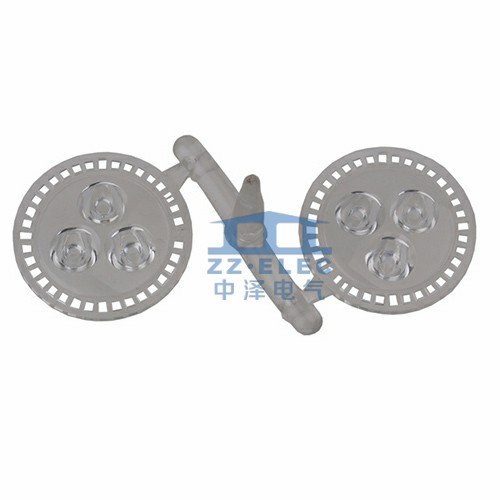# What are the functions of capacitors?

Update:06,Apr,2021
Summary:The role of capacitors:●Coupling: The capacitor used in the coupling circuit is ...
The role of capacitors:
●Coupling: The capacitor used in the coupling circuit is called the coupling capacitor. This type of capacitor circuit is widely used in the resistance-capacitance coupling amplifier and other capacitive coupling circuits to block DC and AC.
●Filtering: The capacitor used in the filter circuit is called the filter capacitor. This capacitor circuit is used in the power supply filter and various filter circuits. The filter capacitor removes the signal in a certain frequency band from the total signal. To
●Decoupling: The capacitor used in the decoupling circuit is called the decoupling capacitor. This capacitor circuit is used in the DC voltage supply circuit of the multi-stage amplifier. The decoupling capacitor eliminates harmful low-frequency cross-connections between each amplifier. To
●High frequency damping: the capacitor used in the high frequency damping circuit is called the high frequency damping capacitor. In the audio negative feedback amplifier, in order to eliminate the high frequency self-excitation that may occur, the capacitor circuit is used to eliminate Possible high-frequency howling from the amplifier.
●Resonance: The capacitor used in the LC resonant circuit is called the resonant capacitor, and this capacitor circuit is required in both the LC parallel and series resonant circuits.
●Bypass: The capacitor used in the bypass circuit is called the bypass capacitor. If you need to remove the signal of a certain frequency band from the signal in the circuit, you can use the bypass capacitor circuit. Depending on the frequency of the removed signal, there is a full frequency domain. (All AC signals) bypass capacitor circuit and high frequency bypass capacitor circuit. To
●Neutralization: The capacitor used in the neutralization circuit is called the neutralization capacitor. In radio high-frequency and intermediate-frequency amplifiers, and TV high-frequency amplifiers, this neutralizing capacitor circuit is used to eliminate self-excitation. To
● Timing: The capacitor used in the timing circuit is called the timing capacitor. Timing capacitor circuits are used in circuits that require time control through capacitor charging and discharging, and capacitors play a role in controlling the size of the time constant. To
●Integration: The capacitor used in the integration circuit is called the integration capacitance. In the synchronous separation circuit of the electric potential field scanning, using this integrating capacitor circuit, the vertical synchronization signal can be taken out from the field composite synchronization signal. To
●Differential: The capacitor used in the differential circuit is called the differential capacitor. In order to obtain the peak trigger signal in the trigger circuit, this differential capacitor circuit is used to obtain the peak pulse trigger signal from various (mainly rectangular pulse) signals.
●Compensation: The capacitor used in the compensation circuit is called the compensation capacitor. In the bass compensation circuit of the deck, this low-frequency compensation capacitor circuit is used to enhance the low-frequency signal in the playback signal. In addition, there are high-frequency compensation capacitors. Circuit. To
Bootstrap: The capacitor used in the bootstrap circuit is called the bootstrap capacitor. The commonly used OTL power amplifier output stage circuit uses this bootstrap capacitor circuit to increase the positive half-cycle amplitude of the signal by a small amount of positive feedback. To
● Frequency division: The capacitor in the frequency division circuit is called the frequency division capacitor. In the speaker frequency division circuit of the speaker, the frequency division capacitor circuit is used to make the high frequency speaker work in the high frequency band, and the mid frequency speaker work in the middle frequency band, and the low frequency The loudspeaker works in the low frequency range.